The OpenFOAM Foundation
sensibleEnthalpy< Thermo > Class Template Reference

Thermodynamics mapping class to expose the sensible enthalpy function as the standard enthalpy function h(T). More...

## Public Member Functions

sensibleEnthalpy ()
Construct. More...

scalar he (const Thermo &thermo, const scalar p, const scalar T) const

scalar cpv (const Thermo &thermo, const scalar p, const scalar T) const

scalar cpBycpv (const Thermo &thermo, const scalar p, const scalar T) const
cp/cp [] More...

scalar HE (const Thermo &thermo, const scalar p, const scalar T) const

scalar THE (const Thermo &thermo, const scalar h, const scalar p, const scalar T0) const
Temperature from sensible enthalpy. More...

## Static Public Member Functions

static word typeName ()
Return the instantiated type name. More...

static word name ()

## Detailed Description

### template<class Thermo> class Foam::sensibleEnthalpy< Thermo >

Thermodynamics mapping class to expose the sensible enthalpy function as the standard enthalpy function h(T).

Definition at line 45 of file sensibleEnthalpy.H.

## Constructor & Destructor Documentation

 sensibleEnthalpy ( )
inline

Construct.

Definition at line 53 of file sensibleEnthalpy.H.

## Member Function Documentation

 static word typeName ( )
inlinestatic

Return the instantiated type name.

Definition at line 60 of file sensibleEnthalpy.H.

 static word name ( )
inlinestatic

Definition at line 67 of file sensibleEnthalpy.H.

References sensibleEnthalpy< Thermo >::he().

Here is the call graph for this function: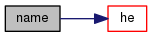scalar he ( const Thermo & thermo, const scalar p, const scalar T ) const
inline

Definition at line 74 of file sensibleEnthalpy.H.

References sensibleEnthalpy< Thermo >::cpv().

Referenced by sensibleEnthalpy< Thermo >::name().

Here is the call graph for this function: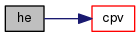Here is the caller graph for this function: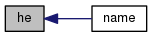scalar cpv ( const Thermo & thermo, const scalar p, const scalar T ) const
inline

Definition at line 85 of file sensibleEnthalpy.H.

References sensibleEnthalpy< Thermo >::cpBycpv().

Referenced by sensibleEnthalpy< Thermo >::he().

Here is the call graph for this function: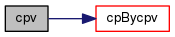Here is the caller graph for this function: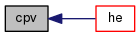scalar cpBycpv ( const Thermo & thermo, const scalar p, const scalar T ) const
inline

cp/cp []

Definition at line 96 of file sensibleEnthalpy.H.

References sensibleEnthalpy< Thermo >::HE().

Referenced by sensibleEnthalpy< Thermo >::cpv().

Here is the call graph for this function: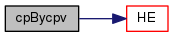Here is the caller graph for this function: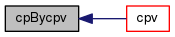scalar HE ( const Thermo & thermo, const scalar p, const scalar T ) const
inline

Definition at line 107 of file sensibleEnthalpy.H.

References sensibleEnthalpy< Thermo >::THE().

Referenced by sensibleEnthalpy< Thermo >::cpBycpv().

Here is the call graph for this function: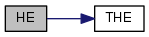Here is the caller graph for this function: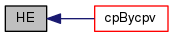scalar THE ( const Thermo & thermo, const scalar h, const scalar p, const scalar T0 ) const
inline

Temperature from sensible enthalpy.

given an initial temperature T0

Definition at line 119 of file sensibleEnthalpy.H.

Referenced by sensibleEnthalpy< Thermo >::HE().

Here is the caller graph for this function: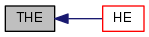The documentation for this class was generated from the following file: